Technical Information Site of Power Supply Design

2017.03.09 Transfer Function

# Slope Transfer Functions: Derivation of Fm in Current Mode

DC/DC Converters: Sharing of Transfer Functions among Control Systems

As slope transfer functions we have derived slope transfer functions for a voltage mode and a current mode, which are representative control methods. Here, we derive a current mode (peak current mode) modulator gain Fm.

When deriving the voltage mode and current mode transfer functions, we explained that a modulator gain Fm is the control voltage change VC governing a change in the duty cycle D. What is derived here is the Fm for the current mode, but the basic approach is the same, and the following should be read while referring to the transfer functions for the voltage mode and current mode.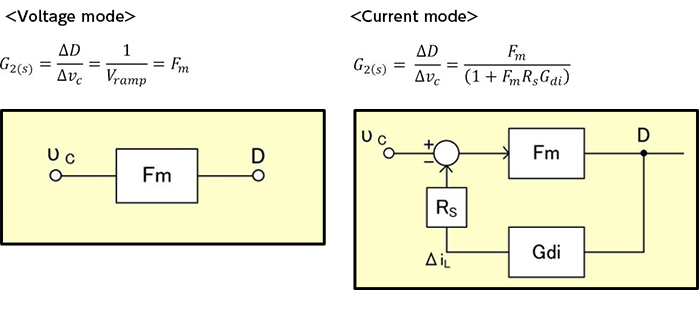Derivation of Fm in current mode

As already explained, coil current information is fed back to the current mode ramp waveform. Hence the ramp waveform when a fixed ramp waveform is not added becomes the feedback component, which is “the ripple current Iripple × the current sense gain RS.”

The switch is turned off where this ramp waveform crosses the error amplifier output VC, and so the coil current information vanishes and the ramp waveform rise stops, becoming zero. This operation is illustrated in Fig. 9.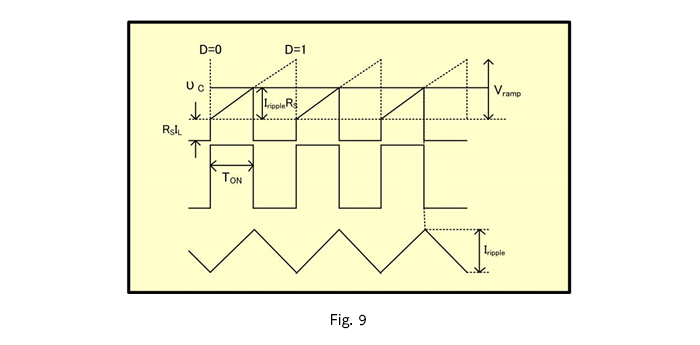Here, the ripple current while turned on can be expressed by equation 3-9.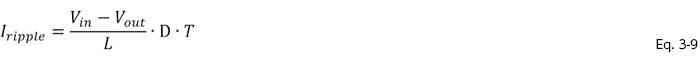In Fig. 9, upon considering similarity of triangles, equation 3-10 results, and upon substituting D for T:TON, equation 3-11 is obtained.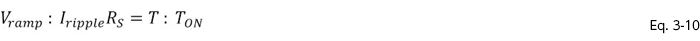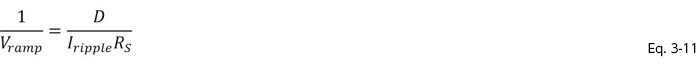Then, substituting equation 3-9 into equation 3-11, Fm can be expressed as equation 3-12.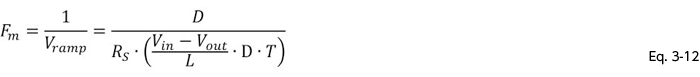Here, if the slope of the ramp waveform due to the coil current is Sn, then Sn can be expressed as in equation 3-13, and the Fm of equation 3-12 can be rewritten as in equation 3-14.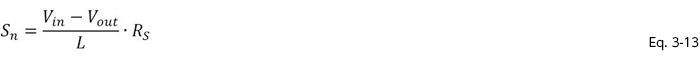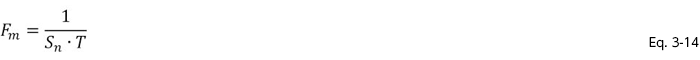Thus Fm for the current mode has been derived.

This website uses cookies.

By continuing to browse this website without changing your web-browser cookie settings, you are agreeing to our use of cookies.